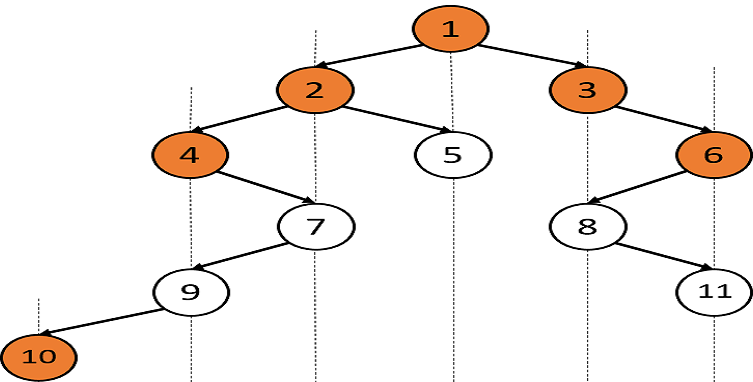# Top View Of Binary Tree

Posted: 19 Oct, 2020
Difficulty: Moderate

## PROBLEM STATEMENT

#### You are given a Binary Tree of integers. You are supposed to return the top view of the given binary tree. The Top view of the binary tree is the set of nodes that are visible when we see the tree from the top.

##### Example:
``````For the given binary tree:
````````````The top view of the tree will be {10, 4, 2, 1, 3, 6}.
``````
##### Input Format:
``````The first line contains an integer 'T' which denotes the number of test cases.

The first line of each test case contains elements of the tree in the level order form. The line consists of values of nodes separated by a single space. In case a node is null, we take -1 in its place.
``````
##### Example:
``````The input for the tree depicted in the below image would be:

1 2 3 4 -1 5 6 -1 7 -1 -1 -1 -1 -1 -1
``````##### Explanation :
``````Level 1 :
The root node of the tree is 1

Level 2 :
Left child of 1 = 2
Right child of 1 = 3

Level 3 :
Left child of 2 = 4
Right child of 2 = null (-1)
Left child of 3 = 5
Right child of 3 = 6

Level 4 :
Left child of 4 = null (-1)
Right child of 4 = 7
Left child of 5 = null (-1)
Right child of 5 = null (-1)
Left child of 6 = null (-1)
Right child of 6 = null (-1)

Level 5 :
Left child of 7 = null (-1)
Right child of 7 = null (-1)

1
2 3
4 -1 5 6
-1 7 -1 -1 -1 -1
-1 -1
``````
##### Note :
``````1. The first not-null node(of the previous level) is treated as the parent of the first two nodes of the current level. The second not-null node (of the previous level) is treated as the parent node for the next two nodes of the current level and so on.

2. The input ends when all nodes at the last level are null(-1).
``````
##### Output Format:
``````For each test case, return the vector/list of all the elements of the top view of the given tree.
``````
##### Note :
``````You don't need to print the output, it has already been taken care of. Just implement the given function.
``````
##### Constraints:
``````1 <= T <= 100
0 <= N <= 1000
0 <= data <= 10^6 and data != -1

Where ‘N’ is the total number of nodes in the binary tree, and 'data' is the value of the binary tree node.

Time limit: 1 sec
``````Approach 1

As we know that all three traversals, i.e. pre-order, in-order and post-order, visit the tree node at once. We can use any of them. Here we are going to use pre-order traversal for the explanation. So while traversing in the pre-order traversal, we will keep track of horizontal distance of the node which is going to be visited from the root node, and we also keep track of the vertical level of that node (where the vertical level of root node would be ‘0’ and its children would be ‘1’ and so on.... ). We will be using the Map which stores the horizontal distance of the node from the root as the key and value in the map maintains a pair of containing the value of the node and the level of the node.

The steps are as follows:

1. Make a Map like:
• map <int, pair<int, int> > visited, where the key of ‘visited’ defines the horizontal distance and value in the map maintains a pair of containing the value of the node and the level of the node.
2. And now call preOrder function. Here, ‘hDistance’ defines the horizontal distance and level defines the depth of the tree.
``applyPreorder(root , hDistance, level,visited) = { applyPreorder(root->left , hDistance-1, level+1,visited) , applyPreorder(root->right , hDistance+1, level+1,visited )}``

3. For every ‘hDistance’ check whether it is visited or not? If it is not visited, then make it visited with the value of node and ‘level’ and if it is already visited, then check if the previous stored ‘level’ is greater than then the current level. If yes, then store the current node because the previous node now is hidden by the current node because the current node has a lesser height.

4. Once we are done with pre-order, our map contains ‘hDistance’ as the key and value corresponding to each ‘hDistance’ stores the pair of nodes and their level that are visible from the top of the tree at that ‘hDistance’. So iterate over the map and store the value of the node in array/list. Let’s say ‘topView’.

5. Return the ‘topView’.